HLIBpro  2.4
Spectrum of Graph Laplacian
Note
The example is based on the Diploma thesis "Effiziente, approximative Berechnung des Spektrums von Graphen mittels hierarchischer Matrizen" von Jan Nitzschmann.

# Problem Description

Given is a Graph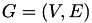with nodes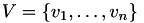and edges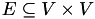. Let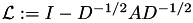be the normalised Laplacian of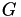with diagonal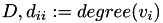, and adjacency matrix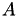,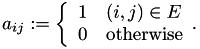We are looking for the spectrum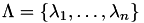of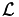. Please note, that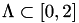with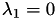.

Computing all eigenvalues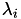is usually extremly costly. However, sometimes not the exact spectrum is of interest but the general distribution of eigenvalues, which permits to characterise certain properties of matrices and especially graphs.

Applying the convolution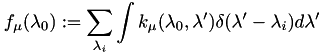with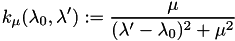and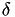being the usual delta distribution, one obtains a graphical representation of the spectrum. An example for this is shown in the following picture for a small graph (left) and the convoluted spectrum of its Laplacian together with the eigenvalues.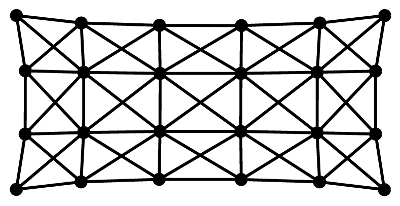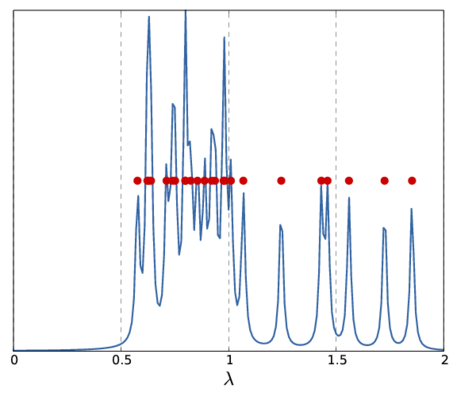Graph Spectrum

Note, that the above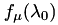only represents a single sample for the whole spectrum. Depending on the sample frequency and the choice of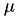, the final image shows more or less details of the spectrum. The following picture show two other versions of the above spectrum with a coarse (left) and fine (right) sampling.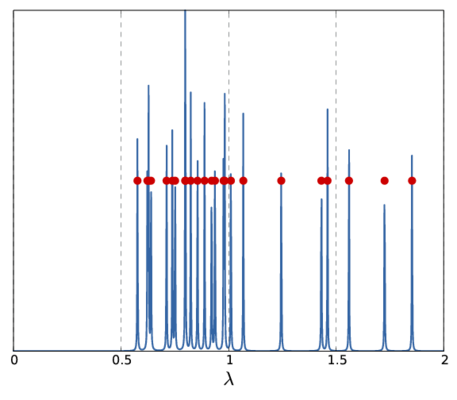# Computation of the Spectrum

The above definition ofcontains the eigenvalues, which are not available. Hence, another way to computeis needed.

We start with the computation of the determinant of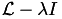using LU factorisation. Since the determinant of triangular matrices is the product of their diagonal entries and since the diagonal entries of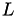are one, this can be restricted to the diagonal entries of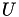: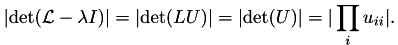Applying the logarithm to both sides of the equation, we can change the product into a sum: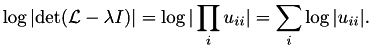Replacing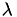by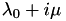, one obtainsHere we have used the identity of the determinant with the corresponding characteristic polynomial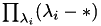.

Finally, differentiation with respect toyieldsReplacing the differentation by a (central) difference quotient makes the problem computable with a series of LU factorisations for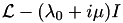for the different values of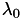. Furthermore, instead of an exact LU decomposition, the H-LU factorisation is used to obtain log-linear costs.

# Sparse to 𝓗-Matrix Conversion

The program starts by converting a sparse matrix, which is assumed to represent a graph laplacian, into an 𝓗-matrix:

#include <iostream>
using namespace std;
using namespace HLIB;
int
main ( int argc,
char ** argv )
{
std::string matrixfile = "graph.mat";
double mu = 1e-2;
double d = 1e-3;
uint nsteps = 100;
double eps = 1e-4;
try
{
HLIB::INIT();
auto M = read_matrix( matrixfile.c_str() );
if ( ! IS_TYPE( M, TSparseMatrix ) )
{
std::cout << "input matrix is not sparse" << std::endl;
return 1;
}
auto S = ptrcast( M.get(), TSparseMatrix * );
S->set_unsymmetric();
TMETISAlgPartStrat part_strat;
TAlgCTBuilder base_ct_builder( & part_strat, 100 );
TAlgNDCTBuilder nd_ct_builder( & base_ct_builder );
auto ct = nd_ct_builder.build( S );
TBCBuilder bct_builder;
auto bct = bct_builder.build( ct.get(), ct.get(), & adm_cond );
TSparseMBuilder h_builder( S, ct->perm_i2e(), ct->perm_e2i() );
auto L = h_builder.build( bct->root(), acc_exact );

In addition toalso the identity matrix is needed to compute:

auto Id = identity( bct.get() );
L->set_complex( true );
Id->set_complex( true );

Both matrices need to be complex valued sinceis, except for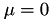, which represents the limit case with exact representation of the spectrum.

# Computing the Difference Quotient

For the computation of the spectrum in the sample space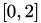equally distant sample points are chosen as defined by the number of steps nsteps, i.e.,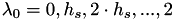with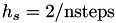.

Afterwards, for each value ofthe difference quotient is computed andas well as the result of the difference quotient are stored:

const auto lambda_0 = i * stepwidth;
const auto f_mu_plus_h = calc_abs_det( L, Id, lambda_0, mu+h, eps );
const auto f_mu_minus_h = calc_abs_det( L, Id, lambda_0, mu-h, eps );
x(i) = lambda_0;
y(i) = (f_mu_plus_h - f_mu_minus_h) / (2 * h);

Since computation for different values ofare independent, they may be computed in parallel. This is done by using the parallel_for algorithm from TBB:

tbb::parallel_for( uint(0), nsteps+1,
[=,&x,&y] ( const uint i )
{
...
} );
Remarks
Although H-LU scales very good with the number of cores, it is even more efficient to perform factorisations is parallel since there are still some portions of the algorithm implemented sequentially.

The result of the computation is stored in two objects of type BLAS::Vector and returned as a std::pair, which completes the function calc_y:

std::pair< BLAS::Vector< double >,
BLAS::Vector< double > >
calc_y ( const TMatrix * L,
const TMatrix * Id,
const double mu,
const double h,
const uint nsteps,
const double eps )
{
BLAS::Vector< double > x( nsteps+1 );
BLAS::Vector< double > y( nsteps+1 );
const double stepwidth = 2.0 / double(nsteps);
tbb::parallel_for( uint(0), nsteps+1,
[=,&x,&y] ( const uint i )
{
const auto lambda_0 = i * stepwidth;
const auto f_mu_plus_h = calc_abs_det( L, Id, lambda_0, mu+h, eps );
const auto f_mu_minus_h = calc_abs_det( L, Id, lambda_0, mu-h, eps );
x(i) = lambda_0;
y(i) = (f_mu_plus_h - f_mu_minus_h) / (2 * h);
} );
return std::pair< BLAS::Vector< double >,
BLAS::Vector< double > >( std::move( x ),
std::move( y ) );
}

This is then called from the main function via

auto spectrum = calc_y( L.get(), Id.get(), mu, d, nsteps, eps );

For visualization, both arrays are written to files in Matlab format, which finishes the program:

TMatlabVectorIO vio;
vio.write( spectrum.first, "x.mat", "x" );
vio.write( spectrum.second, "y.mat", "y" );
HLIB::DONE();
}
catch ( std::exception & e )
{
std::cout << e.what() << std::endl;
}
}

# Computing the Determinant

Left open is the computation of the determinant ofimplemented in calc_abs_det via 𝓗-LU factorisation.

Before the factorisation, the matrix first has to be computed:

double
calc_abs_det ( const TMatrix * L,
const TMatrix * Id,
const double lambda_0,
const double mu,
const double eps )
{
auto L_lmu_Id = L->copy();
add( HLIB::complex( -lambda_0, -mu ), Id, HLIB::complex( 1, 0 ), L_lmu_Id.get(), acc_exact );

By default, 𝓗𝖫𝖨𝖡𝗉𝗋𝗈 uses block-wise LU factorisation, i.e., computes the inverse of diagonal blocks. In this case however, the point-wise LU factorisation is needed since the diagonal entries are used.

fac_options_t opts;
opts.eval = point_wise;
factorise( L_lmu_Id.get(), fixed_prec( eps ), opts );

After the factorisation, these diagonal entries only need to be extracted from the matrix. Note, that L_lmu_id storesandbut since the diagonal entries ofare one and therefore not explicitly stored, the diagonal of L_lmu_id is equal to the diagonal of.

To obtain the diagonal entries the function diagonal is available, which returns a vector containing the coefficients.

In order to prevent stability issues, the function tests for very small values and aborts further computation if such values are present:

auto diag = diagonal( L_lmu_Id.get() );
double sum = 0.0;
for ( auto i : diag->is() )
{
const auto u_ii = diag->centry( i );
const auto norm_uii = std::sqrt( norm( u_ii ) );
if ( norm_uii < 1e-12 )
{
std::cout << "detected near zero entry" << std::endl;
return 0;
}
else
sum += std::log( norm_uii );
}
return sum;
}

# The Plain Program

#include <iostream>
#include <tbb/parallel_for.h>
#include "hlib.hh"
using namespace std;
using namespace HLIB;
double
calc_abs_det ( const TMatrix * L,
const TMatrix * Id,
const double lambda_0,
const double mu,
const double eps )
{
auto L_lmu_Id = L->copy();
opts.eval = point_wise;
add( HLIB::complex( -lambda_0, -mu ), Id, HLIB::complex( 1, 0 ), L_lmu_Id.get(), acc_exact );
factorise( L_lmu_Id.get(), fixed_prec( eps ), opts );
auto diag = diagonal( L_lmu_Id.get() );
double sum = 0.0;
for ( auto i : diag->is() )
{
const auto u_ii = diag->centry( i );
const auto norm_uii = std::sqrt( norm( u_ii ) );
if ( norm_uii < 1e-12 )
{
std::cout << "detected near zero entry" << std::endl;
return 0;
}
else
sum += std::log( norm_uii );
}
return sum;
}
std::pair< BLAS::Vector< double >,
calc_y ( const TMatrix * L,
const TMatrix * Id,
const double mu,
const double d,
const uint nsteps,
const double eps )
{
BLAS::Vector< double > x( nsteps+1 );
BLAS::Vector< double > y( nsteps+1 );
const double stepwidth = 2.0 / double(nsteps);
tbb::parallel_for( uint(0), nsteps+1,
[=,&x,&y] ( const uint i )
{
const auto lambda_0 = i * stepwidth;
const auto f_mu_plus_d = calc_abs_det( L, Id, lambda_0, mu+d, eps );
const auto f_mu_minus_d = calc_abs_det( L, Id, lambda_0, mu-d, eps );
x(i) = lambda_0;
y(i) = (f_mu_plus_d - f_mu_minus_d) / (2 * d);
} );
return std::pair< BLAS::Vector< double >,
BLAS::Vector< double > >( std::move( x ),
std::move( y ) );
}
int
main ( int argc,
char ** argv )
{
std::string matrixfile = "graph.mat";
double mu = 1e-2;
double h = 1e-3;
uint nsteps = 100;
double eps = 1e-4;
try
{
HLIB::INIT();
auto M = read_matrix( matrixfile.c_str() );
if ( ! IS_TYPE( M, TSparseMatrix ) )
{
std::cout << "input matrix is not sparse" << std::endl;
return 1;
}
auto S = ptrcast( M.get(), TSparseMatrix * );
S->set_unsymmetric();
TMETISAlgPartStrat part_strat;
TAlgCTBuilder base_ct_builder( & part_strat, 100 );
TAlgNDCTBuilder nd_ct_builder( & base_ct_builder );
auto ct = nd_ct_builder.build( S );
TBCBuilder bct_builder;
auto bct = bct_builder.build( ct.get(), ct.get(), & adm_cond );
TSparseMBuilder h_builder( S, ct->perm_i2e(), ct->perm_e2i() );
auto L = h_builder.build( bct->root(), acc_exact );
auto Id = identity( bct.get() );
L->set_complex( true );
Id->set_complex( true );
auto spectrum = calc_y( L.get(), Id.get(), mu, h, nsteps, eps );
vio.write( spectrum.first, "x.mat", "x" );
vio.write( spectrum.second, "y.mat", "y" );
HLIB::DONE();
}
catch ( std::exception & e )
{
std::cout << e.what() << std::endl;
}
}

# Example Matrices

The Sparse Matrix Collection from the University of Florida provides a wide range of sparse matrices.

The following Matlab function will compute the normalised Laplacian from a given sparse matrix, assuming the matrix contains the adjacency matrix of the graph (loops are ignored!).

function [ Ln ] = laplacian ( A )
s = size(A);
n = s(1);
sums = sum( A );
D=sparse([1:n],[1:n],sums,n,n);
L=tril(A,-1)+D+triu(A,1);
invsums = 1./sqrt(sums);
D=sparse([1:n],[1:n],invsums,n,n);
Ln = D*L*D;
end